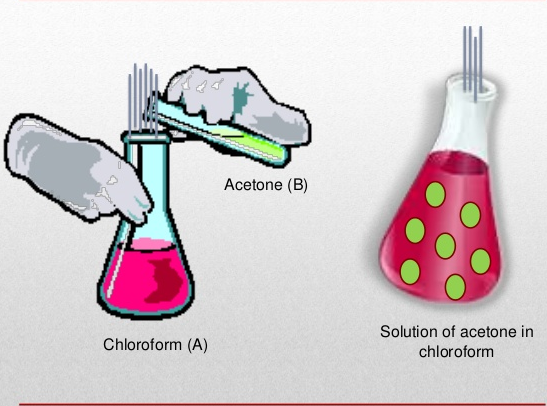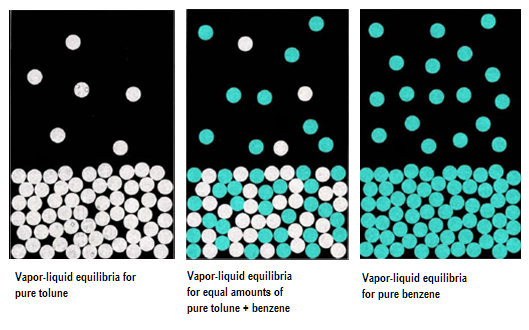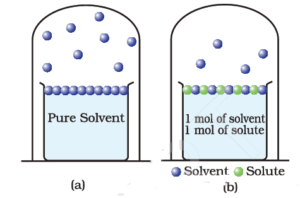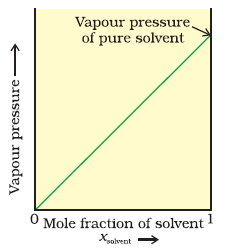×#### Thank you for registering.

One of our academic counsellors will contact you within 1 working day.

Click to Chat

1800-1023-196

+91-120-4616500

CART 0

• 0

MY CART (5)

Use Coupon: CART20 and get 20% off on all online Study Material

ITEM
DETAILS
MRP
DISCOUNT
FINAL PRICE
Total Price: Rs.

There are no items in this cart.
Continue Shopping```Vapour Pressure of Liquid Solutions

Vapour Pressure of Liquid Solutions

Vapour Pressure of Liquid in Liquid Solution

Raoult’s Law

Conclusions on Vapour Pressure relation in Liquid-Liquid Solution

Vapour Pressure of Solid in Liquid Solution

Vapour Pressure of Liquid SolutionsImage 1: Example of liquid in liquid solution

Liquid Solution is formed when we dissolve a solid, liquid or gas in a particular liquid solvent. Vapour Pressure of liquid solutions is defined as the pressure exerted by the vapours on the liquid solvent when kept in equilibrium and a certain temperature. It varies with the nature of liquid and temperature of the surroundings. Some characteristics of the vapour pressure of liquid solutions are as follows:

The pure liquid has more vapour pressure as compared to liquid’s solution. For Example, take two beaker one filled with water and other with lemon juice and water, you’ll find that the beaker filled with water has more vapour pressure

Vapour pressure is inversely proportional to forces of attraction between molecules of a liquid

Vapour pressure increases with increase in temperature, as molecules gain kinetic energy and vapourise brisklyImage 2: Vapour pressure equilibrium in different solutions

Liquid solution can contain volatile solute and solvent as well. In most cases, the solvent is volatile in nature and the solute left out to be non-volatile. Vapour pressure can be evaluated in two cases which are:

Vapour pressure of liquid-liquid solutionsthat is, solute and solvent both are in liquid phase

Vapour pressure of solid- liquid solutions that is, solute is in solid state and solvent is in liquid phase

Vapour Pressure of Liquid-Liquid Solutions

To find out vapour pressure of the liquid-liquid solution, we take two volatile liquid solutions and name their liquid component as A and B. When we place the volatile liquid with their components in a closed vessel, we find that equilibrium is established between the liquid phase and vapour phase.

Suppose Ptotal is the overall vapour pressure at an equilibrium state and let PA and PB be the partial vapour pressures of components A and B respectively. Adding further, the mole fraction of respective components is xA and xB respectively.

To evaluate vapour pressure of volatile liquids, we use Raoult’s Law.

Raoult’s Law

In 1986, a French Chemist named Francois Marte Raoult proposed a quantitative relation between partial pressure and mole fraction of volatile liquids. The law states that mole fraction of the solute component is directly proportional to its partial pressure.

So, according to Raoult’s Law, the partial pressure of A will be

PA ∝ xA

PA = PA0 xA

where PA0 is the vapour pressure of pure liquid component A. Similarly partial pressure of B will be

PB ∝ xB

PB = PB0 xB

where PB0 is the vapour pressure of pure liquid component B.

Now, by Dalton’s law of partial pressures, this states that total pressure ( Ptotal) the solution placed in a container is the sum of partial pressures of its respective components. That is

Ptotal = PA + PB

Ptotal = PA0 xA + PB0 xB

Also since, xA + xB = 1 the relation can also be re-written as

Ptotal = PA0 + (PB0 - PA0) xBImage 3: Graph between mole fraction and vapour pressure in liquid-liquid solution

Conclusions on Vapour pressure relation in liquid-liquid solutions

Following conclusions are observed from the following relation.

Total vapour pressure relies on mole fraction of any one of the component that is, either A or B. For Example, the equation can also be written as

Ptotal = PB0 + (PA0 – PB0) XA

We can draw a linear graph between total pressure and mole fraction of any of the following component

Total vapour pressure increases or decreases with respect to mole fraction of component A, depending on pure vapour pressures of components A and B

If the components are in vapour form, the total vapour pressure can be calculated as

PA = yA Ptotal

PB = yB Ptotal

where yA and yB are mole fraction of components A and B in the vapour phase.

Example: The vapour pressures of pure chloroform CHCl3 and dichloromethane CH2Cl2 are 200 mm Hg and 415 mm Hg. If we add 30 g of CHCl3 and 42 g of CH2Cl2 at some normal temperature 298 K, then what will be the total vapour pressure of solution? Also, find out mole fraction of both the components in the vapour phase.

So, in this problem, we have two liquid solutions chloroform ( CHCl3) and dichloromethane CH2Cl2. To find out total vapour pressure, we need mole fraction of both the components.

We know that mole fraction of component A = moles of A / Total moles of the solution

And moles of component is mass of component/ molar mass of component

Moles of chloroform CHCl3 = 30/ 119.5 = 0.25

Moles of dichloromethane CH2Cl2 = 42/ 85 = 0.49

Let A component be CHCl3 and B component be CH2Cl2 , then

xA = 0.25 / ( 0.25 + 0.49) = 0.25 / 0.74 = 0.33

xB = 0.49 / ( 0.25 + 0.49) = 0.49 / 0.74 = 0.66

Also, we have PA0 as 200 mm Hg and PB0 as 415 mm Hg

Then total vapour pressure P equals

Ptotal = PA0 xA + PB0 xB

Ptotal = 200 × 0.33 + 415 × 0.66

Ptotal = 339.9 mm Hg

Let the mole fraction of CHCl3 chloroform yA and CH2Cl2 be yB in vapour phase, then we know that

PA = yA Ptotal

PA0 xA = yA Ptotal

200 × 0.33 = yA Ptotal

66 / 339.9 = yA

0.19 = yA

Also, yA + yB = 1

Then yB = 1 – yA = 1 – 0.19 = 0.81

So, the total vapour pressure of the solution is 339.3 mm Hg and mole fraction of CHCl3 chloroform is 0.19 and that of CH2Cl2 dichloromethane is 0.81 respectively.

Vapour Pressure of Solutions of Solids in Liquids

Another type of solutions is solids in liquid solution, in which we take solid as the solute and liquid as the solvent. For example, when we dissolve glucose, sugar or salt in water, we get solids in liquids type of solution. Generally, the solute is non-volatile in nature and the vapour pressure is less than the pure vapour pressure of the solution.

Why Vapour Pressure decreases when we add a non-volatile solute in the solvent?

The decrease in vapour pressure is due to:

As evaporation is a surface phenomenon, the more the surface, the greater the evaporation and hence more the vapour pressure. In a pure liquid, there is more surface area available for the molecules to vapourise, thereby have more vapour pressure. On the other hand, when we add a non-volatile solute, the solvent molecules get less surface to escape and hence experience low vapour pressure

The number of molecules evaporating or leaving the surface is much greater in pure liquid solutions to that of non-volatile solute in solventImage 4: Pure solvent has more number of molecules on the surface as compared to non-volatile solute- solvent solution

To find out overall vapour pressure of the solid-liquid solution, we consider a solution in which lets say A is solvent and B is solute. According to Raoult’s law, we know that partial vapour pressure of individual component (solute/solvent) is directly proportional to its mole fraction.

Now, when we add a non-volatile solute, it is obvious that vapour pressure will only come from the solvent part, as they are an only available component in the vapour phase. Hence, if PA is the vapour pressure of the solvent, xA is its mole-fraction and PA0 is the vapour pressure of the pure solvent, then by Raoult’s law, the relation will come out to be:

PA ∝ xA

PA = PA0 xAImage 5: Graph between Vapour Pressure and mole fraction in case of solid in liquid solution.

When we plot a graph between mole fraction of solvent and vapour pressure, we find its nature to be linear.

Watch this Video for more reference

Vapour Pressure of Liquid Solution
```### Course Features

• 731 Video Lectures
• Revision Notes
• Previous Year Papers
• Mind Map
• Study Planner
• NCERT Solutions
• Discussion Forum
• Test paper with Video Solution